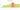# د.ج.‏

## Algerian Dinar

Algeria's national currency, the dinar, is divisible into 100 centimes. Due to their incredibly low worth, centimes are now considered outdated.

#### how has the value of the currency changed in the last year?## USD 1 = DZD 134.2

How much is10 US dollars worth inAlgerian dinars?
At the current exchange rate, 10 US dollars is worth 1,342.04 Algerian dinars
How much is50 US dollars worth inAlgerian dinars?
At the current exchange rate, 50 US dollars is worth 6,710.18 Algerian dinars
How much is100 US dollars worth inAlgerian dinars?
At the current exchange rate, 100 US dollars is worth 13,420.35 Algerian dinars
How much is500 US dollars worth inAlgerian dinars?
At the current exchange rate, 500 US dollars is worth 67,101.76 Algerian dinars
How much is2,000 US dollars worth inAlgerian dinars?
At the current exchange rate, 2,000 US dollars is worth 268,407.03 Algerian dinars
How much is10 US dollars worth inAlgerian dinars?
At the current exchange rate, 10 US dollars is worth 1,342.04 Algerian dinars
How much is50 US dollars worth inAlgerian dinars?
At the current exchange rate, 50 US dollars is worth 6,710.18 Algerian dinars
How much is100 US dollars worth inAlgerian dinars?
At the current exchange rate, 100 US dollars is worth 13,420.35 Algerian dinars
How much is500 US dollars worth inAlgerian dinars?
At the current exchange rate, 500 US dollars is worth 67,101.76 Algerian dinars
How much is2,000 US dollars worth inAlgerian dinars?
At the current exchange rate, 2,000 US dollars is worth 268,407.03 Algerian dinars